# Background

FFD estimates are based on the number of days between 50th, 80th, and 90th percentiles of last spring frost day and 50th, 20th, and 10th percentiles of first fall frost day. FFD values are associated with 50%, 80%, or 90% confidence of being within the frost-free period.

# Examples

## Setup R Environment

With a recent version of R (>= 2.15), it is possible to get all of the packages that this tutorial depends on via:

# run these commands in the R console
install.packages('sharpshootR', dep=TRUE)
install.packages('soilDB', dep=TRUE)
install.packages('plyr', dep=TRUE)
install.packages('daymetr', dep=TRUE)

install.packages('devtools', dep=TRUE)
devtools::install_github("ncss-tech/sharpshootR", dependencies=FALSE, upgrade_dependencies=FALSE)

library(sharpshootR)
library(soilDB)
library(plyr)

## CDEC

The California Data Exchange Center (CDEC) hosts all kinds of useful climate data. You can get these easily by station and sensor code with the CDECquery() function. See the related tutorial for more ideas.

# HHM: Highland Meadows
# 32: daily min air temperature
x <- CDECquery(id='HHM', sensor=32, interval='D', start='1900-01-01', end='2016-12-31')

# estimate FFD over all years, stored in 'year' column
# air temperature are in deg F
x.ffd <- FFD(x, returnDailyPr = TRUE, frostTemp=32)

# basic summary
kable(x.ffd$summary) ffd.50 ffd.80 ffd.90 spring.50 spring.80 spring.90 fall.50 fall.80 fall.90 n.yrs 92 72 67 164 168 169 256 240 236 10 # graphical summary of FFD estimates par(mar=c(4,3.5,3,1)) FFDplot(x.ffd, 'CDEC - Highland Meadows')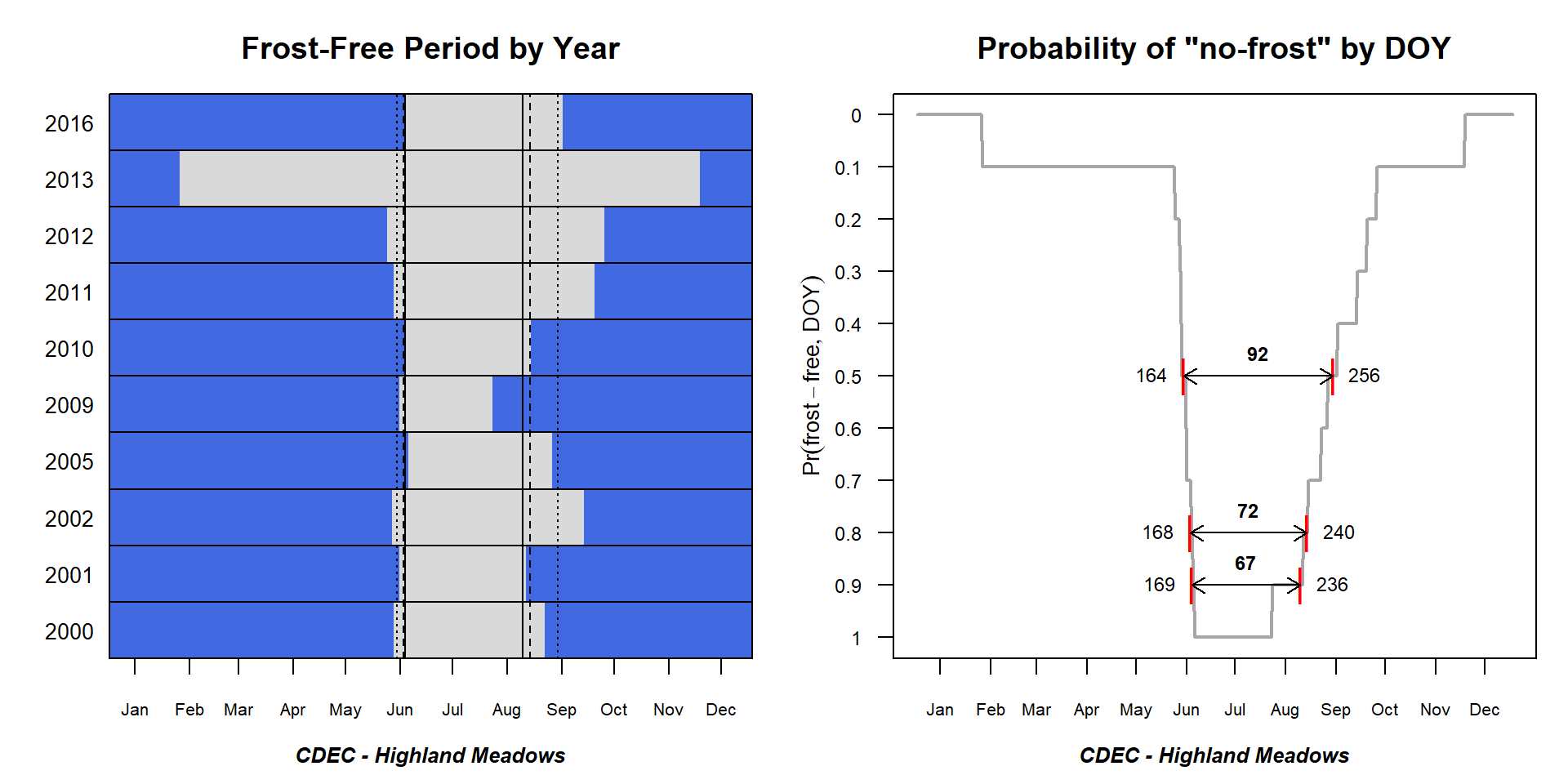## SNOTEL Data from SNOTEL Station 365. # site code and year range are required x <- fetchSCAN(site.code=365, year=c(1995:2016)) # extract the daily min air temperature records x <- x$TMIN

# re-name and add "year" column
names(x) <- 'datetime'
x$year <- as.integer(format(x$datetime, "%Y"))

# estimate FFD over all years
# air temperature are in deg C
x.ffd <- FFD(x, returnDailyPr = TRUE, frostTemp=0)

# basic summary
kable(x.ffd$summary) ffd.50 ffd.80 ffd.90 spring.50 spring.80 spring.90 fall.50 fall.80 fall.90 n.yrs 101 88 75 160 163 170 260 251 244 22 # graphical summary of FFD estimates par(mar=c(4,3.5,3,1)) FFDplot(x.ffd, 'SNOTEL Station 365')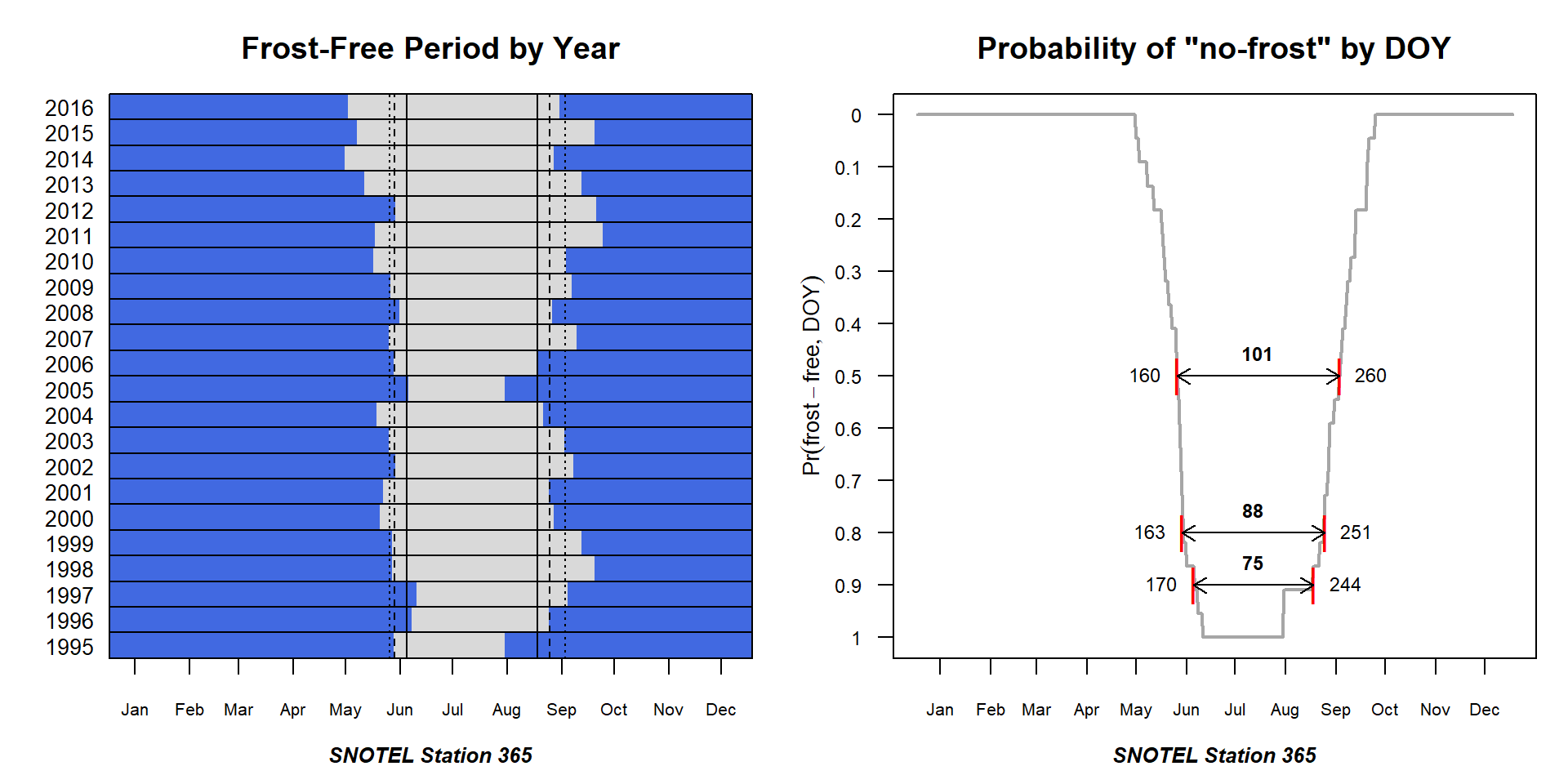## SCAN Data from SCAN Station 808. # same interface as SNOTEL # site code and year range are required x <- fetchSCAN(site.code=808, year=c(1995:2016)) # extract the daily min air temperature records x <- x$TMIN

# re-name and add "year" column
names(x) <- 'datetime'
x$year <- as.integer(format(x$datetime, "%Y"))

# estimate FFD over all years
# air temperature are in deg C
x.ffd <- FFD(x, returnDailyPr = TRUE, frostTemp=0)

# basic summary
kable(x.ffd$summary) ffd.50 ffd.80 ffd.90 spring.50 spring.80 spring.90 fall.50 fall.80 fall.90 n.yrs 127 114 95 142 149 159 268 262 254 22 # graphical summary of FFD estimates par(mar=c(4,3.5,3,1)) FFDplot(x.ffd, 'SCAN Station 808')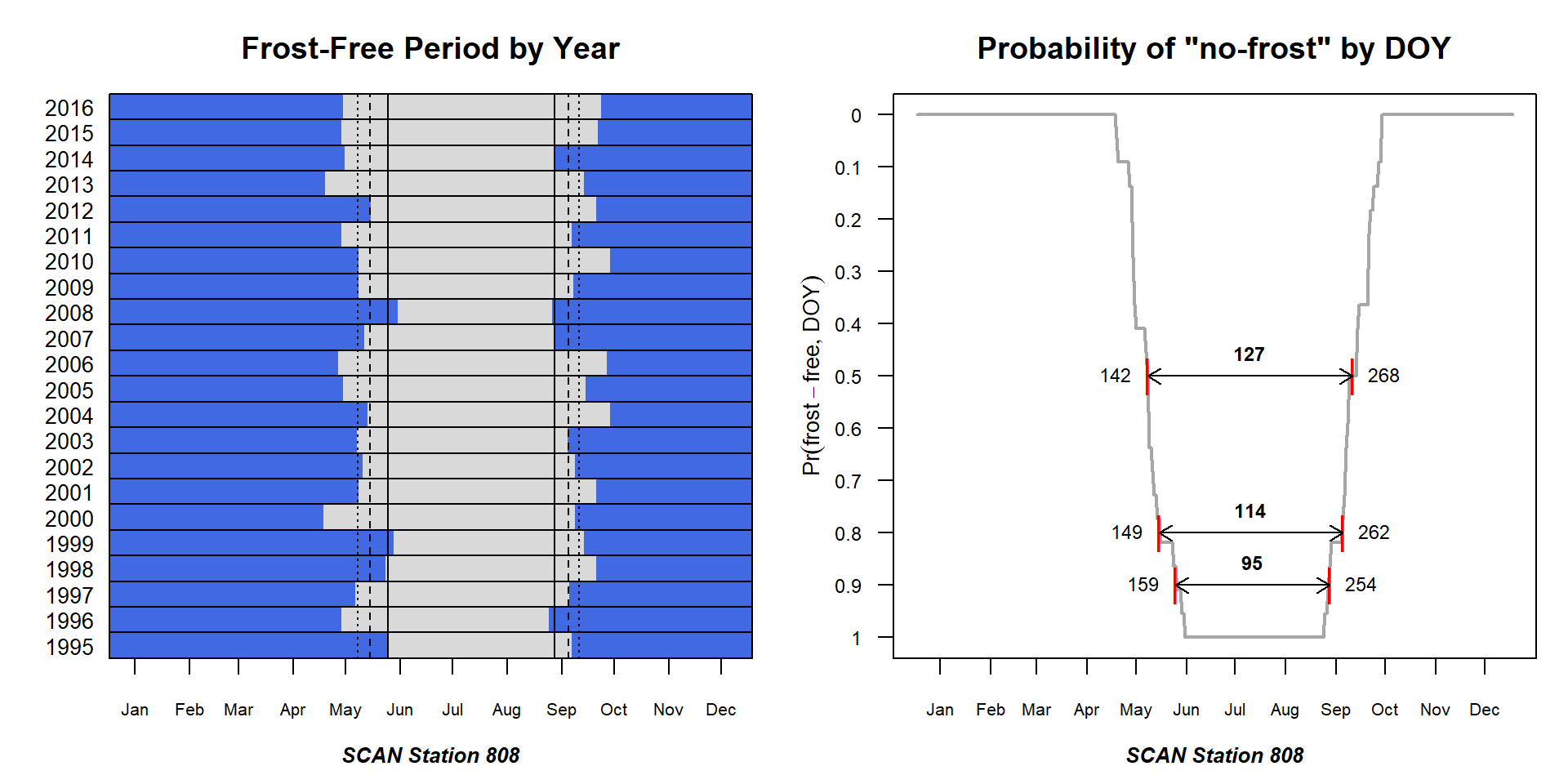## Henry Mount Soil Climate DB Air temperature data associated with NASIS user site ID “1997NV005022”. # must specify gran='hour' for hourly data # otherwise daily mean values are returned x <- fetchHenry(usersiteid = '1997NV005022', what = 'airtemp', gran='hour') # extract air temperature records x <- x$airtemp
# convert date-time stamp to date (truncate time component)
x$datetime <- as.Date(x$date_time)

# compute daily min air temperature
x <- ddply(x, 'datetime', summarize, value=min(sensor_value, na.rm=TRUE))
x$year <- as.integer(format(x$datetime, "%Y"))

# estimate FFD over all years
# air temperature are in deg C
x.ffd <- FFD(x, returnDailyPr = TRUE, frostTemp=0)

# basic summary
kable(x.ffd$summary) ffd.50 ffd.80 ffd.90 spring.50 spring.80 spring.90 fall.50 fall.80 fall.90 n.yrs 100 88 77 164 170 172 265 257 249 10 # graphical summary of FFD estimates par(mar=c(4,3.5,3,1)) FFDplot(x.ffd, 'Henry: 1997NV005022')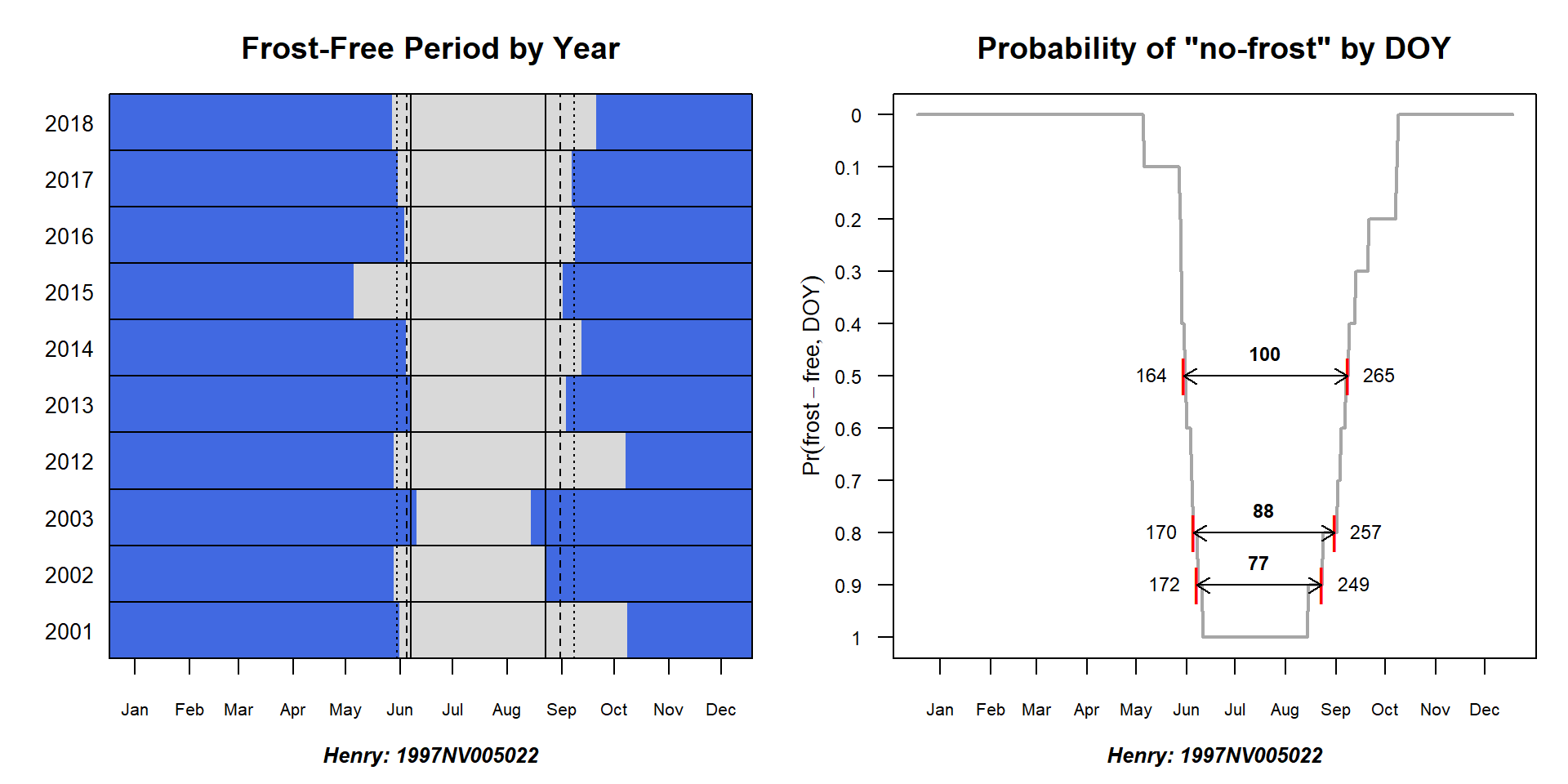## DAYMET Daily minimum air temperature data from DAYMET, via coordinate API at (-120, 38). The daytmetr package greatly simplifies data retrieval. library(daymetr) # convenience function, returns data suitable for FFD eval getDayMet <- function(x, y) { # get 30yr span for single coordinate d <- download_daymet("daymet", lat = y, lon = x, start = 1981, end = 2010, internal = TRUE ) # keep only the data d <- d$data

# format data required for FFD calc
d$datetime <- as.Date(sprintf('%s %s', d$year, d$yday), format="%Y %j") d$value <- d$tmin..deg.c. # note, these are deg C return(d[, c('datetime', 'year', 'value')]) } # get data x <- getDayMet(x = -120, y = 38) # estimate FFD over all years # air temperature are in deg C x.ffd <- FFD(x, returnDailyPr = TRUE, frostTemp=0) # basic summary kable(x.ffd$summary)
ffd.50 ffd.80 ffd.90 spring.50 spring.80 spring.90 fall.50 fall.80 fall.90 n.yrs
166 131 124 132 148 152 298 280 277 30
# graphical summary of FFD estimates
par(mar=c(4,3.5,3,1))
FFDplot(x.ffd, 'DAYMET at (-120, 38)')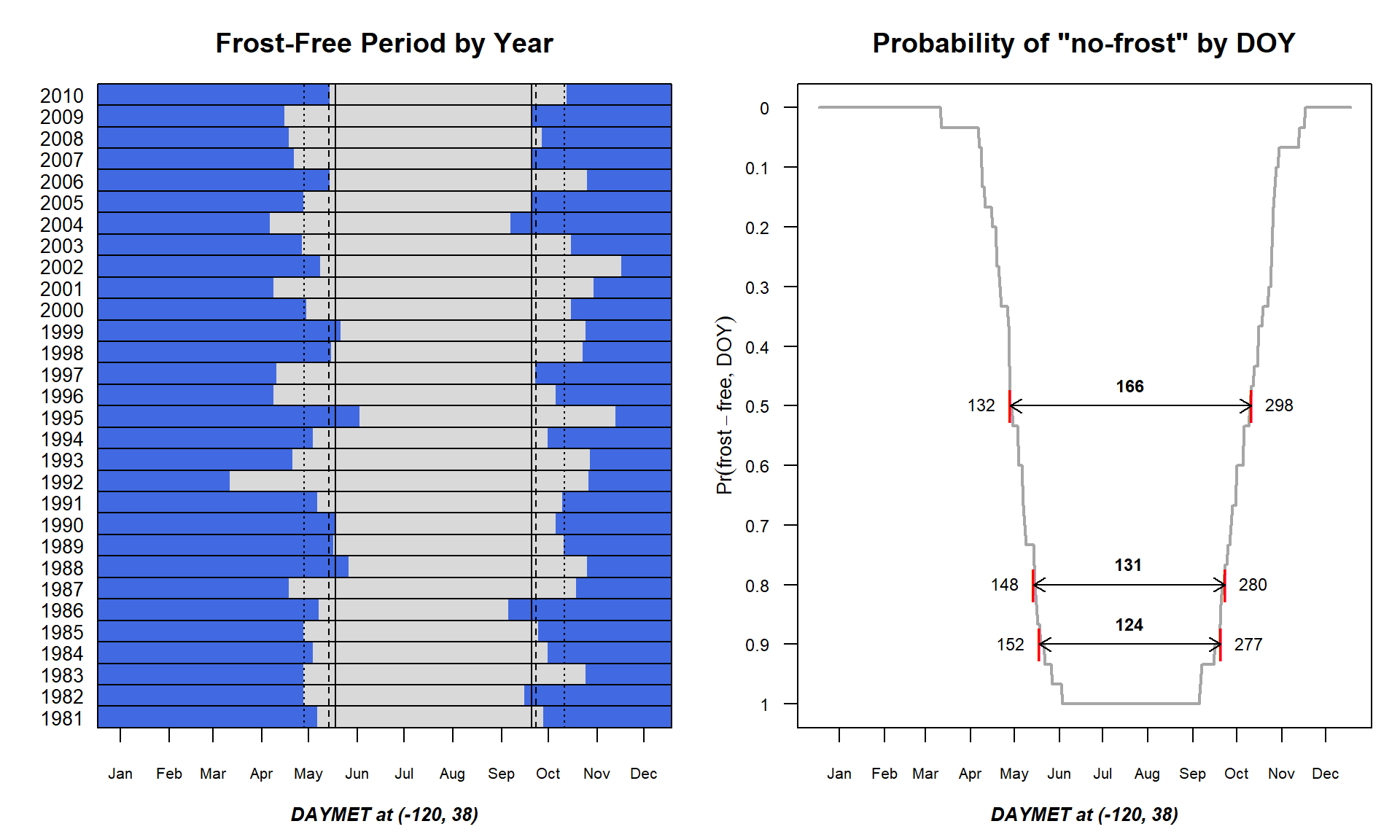This document is based on sharpshootR version 1.7.3 and soilDB version 2.6.1.